Transition Metals and Coordination Chemistry

# 123 Spectroscopic and Magnetic Properties of Coordination Compounds

### Learning Objectives

By the end of this section, you will be able to:

• Outline the basic premise of crystal field theory (CFT)
• Identify molecular geometries associated with various d-orbital splitting patterns
• Predict electron configurations of split d orbitals for selected transition metal atoms or ions
• Explain spectral and magnetic properties in terms of CFT concepts

The behavior of coordination compounds cannot be adequately explained by the same theories used for main group element chemistry. The observed geometries of coordination complexes are not consistent with hybridized orbitals on the central metal overlapping with ligand orbitals, as would be predicted by valence bond theory. The observed colors indicate that the d orbitals often occur at different energy levels rather than all being degenerate, that is, of equal energy, as are the three p orbitals. To explain the stabilities, structures, colors, and magnetic properties of transition metal complexes, a different bonding model has been developed. Just as valence bond theory explains many aspects of bonding in main group chemistry, crystal field theory is useful in understanding and predicting the behavior of transition metal complexes.

### Crystal Field Theory

To explain the observed behavior of transition metal complexes (such as how colors arise), a model involving electrostatic interactions between the electrons from the ligands and the electrons in the unhybridized d orbitals of the central metal atom has been developed. This electrostatic model is crystal field theory (CFT). It allows us to understand, interpret, and predict the colors, magnetic behavior, and some structures of coordination compounds of transition metals.

CFT focuses on the nonbonding electrons on the central metal ion in coordination complexes not on the metal-ligand bonds. Like valence bond theory, CFT tells only part of the story of the behavior of complexes. However, it tells the part that valence bond theory does not. In its pure form, CFT ignores any covalent bonding between ligands and metal ions. Both the ligand and the metal are treated as infinitesimally small point charges.

All electrons are negative, so the electrons donated from the ligands will repel the electrons of the central metal. Let us consider the behavior of the electrons in the unhybridized d orbitals in an octahedral complex. The five d orbitals consist of lobe-shaped regions and are arranged in space, as shown in (Figure). In an octahedral complex, the six ligands coordinate along the axes.

The directional characteristics of the five d orbitals are shown here. The shaded portions indicate the phase of the orbitals. The ligands (L) coordinate along the axes. For clarity, the ligands have been omitted from the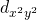orbital so that the axis labels could be shown.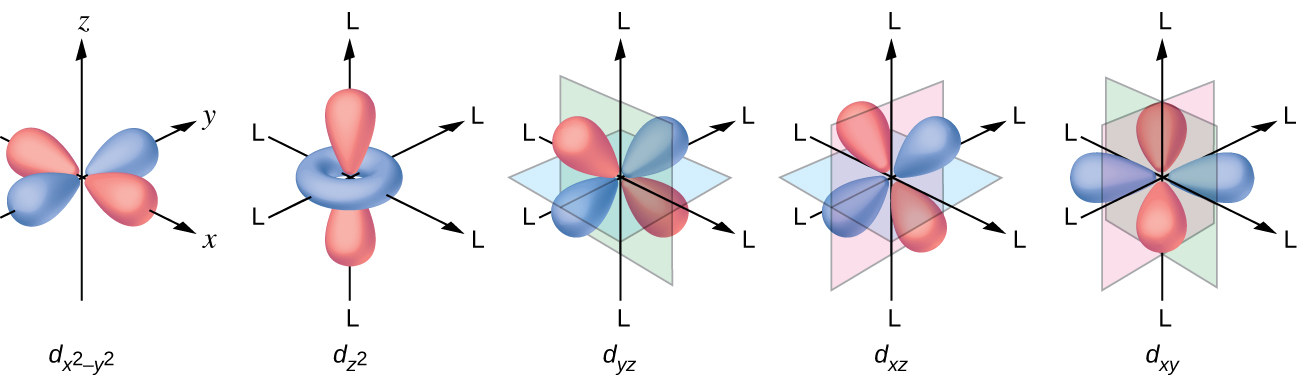In an uncomplexed metal ion in the gas phase, the electrons are distributed among the five d orbitals in accord with Hund’s rule because the orbitals all have the same energy. However, when ligands coordinate to a metal ion, the energies of the d orbitals are no longer the same.

In octahedral complexes, the lobes in two of the five d orbitals, the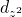andorbitals, point toward the ligands ((Figure)). These two orbitals are called the eg orbitals (the symbol actually refers to the symmetry of the orbitals, but we will use it as a convenient name for these two orbitals in an octahedral complex). The other three orbitals, the dxy, dxz, and dyz orbitals, have lobes that point between the ligands and are called the t2g orbitals (again, the symbol really refers to the symmetry of the orbitals). As six ligands approach the metal ion along the axes of the octahedron, their point charges repel the electrons in the d orbitals of the metal ion. However, the repulsions between the electrons in the eg orbitals (theandorbitals) and the ligands are greater than the repulsions between the electrons in the t2g orbitals (the dzy, dxz, and dyz orbitals) and the ligands. This is because the lobes of the eg orbitals point directly at the ligands, whereas the lobes of the t2g orbitals point between them. Thus, electrons in the eg orbitals of the metal ion in an octahedral complex have higher potential energies than those of electrons in the t2g orbitals. The difference in energy may be represented as shown in (Figure).

In octahedral complexes, the eg orbitals are destabilized (higher in energy) compared to the t2g orbitals because the ligands interact more strongly with the d orbitals at which they are pointed directly.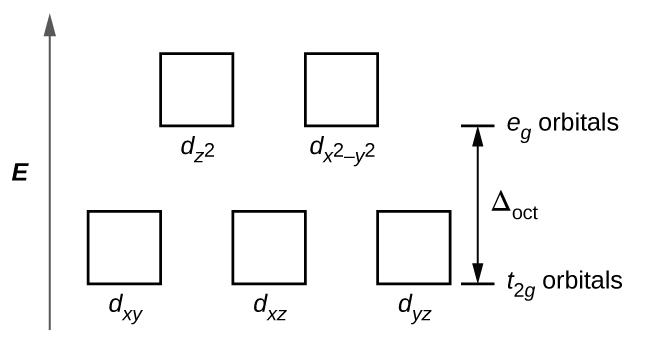The difference in energy between the eg and the t2g orbitals is called the crystal field splitting and is symbolized by Δoct, where oct stands for octahedral.

The magnitude of Δoct depends on many factors, including the nature of the six ligands located around the central metal ion, the charge on the metal, and whether the metal is using 3d, 4d, or 5d orbitals. Different ligands produce different crystal field splittings. The increasing crystal field splitting produced by ligands is expressed in the spectrochemical series, a short version of which is given here: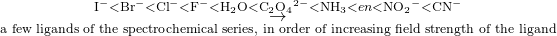In this series, ligands on the left cause small crystal field splittings and are weak-field ligands, whereas those on the right cause larger splittings and are strong-field ligands. Thus, the Δoct value for an octahedral complex with iodide ligands (I) is much smaller than the Δoct value for the same metal with cyanide ligands (CN).

Electrons in the d orbitals follow the aufbau (“filling up”) principle, which says that the orbitals will be filled to give the lowest total energy, just as in main group chemistry. When two electrons occupy the same orbital, the like charges repel each other. The energy needed to pair up two electrons in a single orbital is called the pairing energy (P). Electrons will always singly occupy each orbital in a degenerate set before pairing. P is similar in magnitude to Δoct. When electrons fill the d orbitals, the relative magnitudes of Δoct and P determine which orbitals will be occupied.

In [Fe(CN)6]4−, the strong field of six cyanide ligands produces a large Δoct. Under these conditions, the electrons require less energy to pair than they require to be excited to the eg orbitals (Δoct > P). The six 3d electrons of the Fe2+ ion pair in the three t2g orbitals ((Figure)). Complexes in which the electrons are paired because of the large crystal field splitting are called low-spin complexes because the number of unpaired electrons (spins) is minimized.

Iron(II) complexes have six electrons in the 5d orbitals. In the absence of a crystal field, the orbitals are degenerate. For coordination complexes with strong-field ligands such as [Fe(CN)6]4−, Δoct is greater than P, and the electrons pair in the lower energy t2g orbitals before occupying the eg orbitals. With weak-field ligands such as H2O, the ligand field splitting is less than the pairing energy, Δoct less than P, so the electrons occupy all d orbitals singly before any pairing occurs.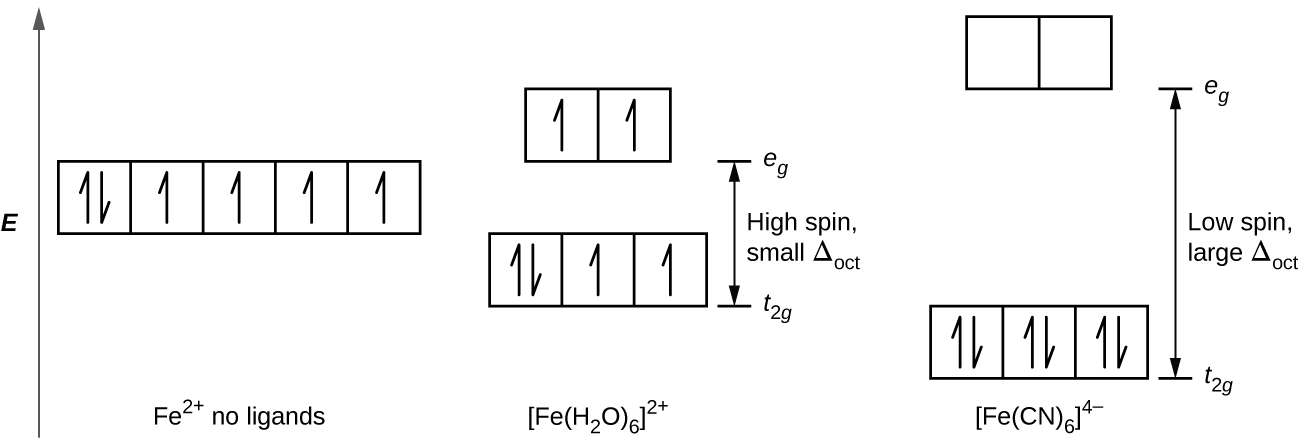In [Fe(H2O)6]2+, on the other hand, the weak field of the water molecules produces only a small crystal field splitting (Δoct < P). Because it requires less energy for the electrons to occupy the eg orbitals than to pair together, there will be an electron in each of the five 3d orbitals before pairing occurs. For the six d electrons on the iron(II) center in [Fe(H2O)6]2+, there will be one pair of electrons and four unpaired electrons ((Figure)). Complexes such as the [Fe(H2O)6]2+ ion, in which the electrons are unpaired because the crystal field splitting is not large enough to cause them to pair, are called high-spin complexes because the number of unpaired electrons (spins) is maximized.

A similar line of reasoning shows why the [Fe(CN)6]3− ion is a low-spin complex with only one unpaired electron, whereas both the [Fe(H2O)6]3+ and [FeF6]3− ions are high-spin complexes with five unpaired electrons.

High- and Low-Spin Complexes Predict the number of unpaired electrons.

(a) K3[CrI6]

(b) [Cu(en)2(H2O)2]Cl2

(c) Na3[Co(NO2)6]

Solution The complexes are octahedral.

(a) Cr3+ has a d3 configuration. These electrons will all be unpaired.

(b) Cu2+ is d9, so there will be one unpaired electron.

(c) Co3+ has d6 valence electrons, so the crystal field splitting will determine how many are paired. Nitrite is a strong-field ligand, so the complex will be low spin. Six electrons will go in the t2g orbitals, leaving 0 unpaired.

Check Your Learning The size of the crystal field splitting only influences the arrangement of electrons when there is a choice between pairing electrons and filling the higher-energy orbitals. For which d-electron configurations will there be a difference between high- and low-spin configurations in octahedral complexes?

Answer:

d4, d5, d6, and d7

CFT for Other Geometries CFT is applicable to molecules in geometries other than octahedral. In octahedral complexes, remember that the lobes of the eg set point directly at the ligands. For tetrahedral complexes, the d orbitals remain in place, but now we have only four ligands located between the axes ((Figure)). None of the orbitals points directly at the tetrahedral ligands. However, the eg set (along the Cartesian axes) overlaps with the ligands less than does the t2g set. By analogy with the octahedral case, predict the energy diagram for the d orbitals in a tetrahedral crystal field. To avoid confusion, the octahedral eg set becomes a tetrahedral e set, and the octahedral t2g set becomes a t2 set.

This diagram shows the orientation of the tetrahedral ligands with respect to the axis system for the orbitals.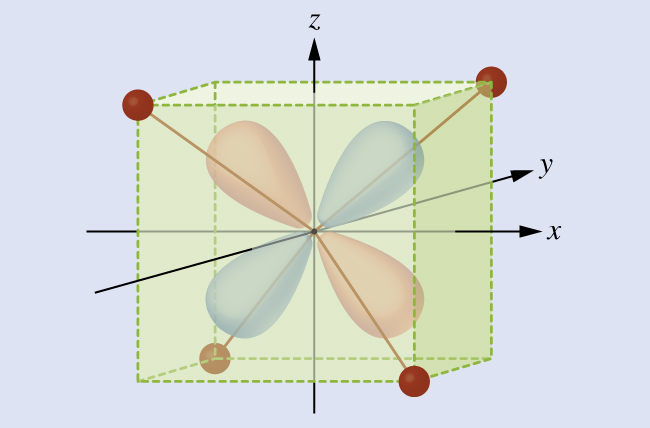Solution Since CFT is based on electrostatic repulsion, the orbitals closer to the ligands will be destabilized and raised in energy relative to the other set of orbitals. The splitting is less than for octahedral complexes because the overlap is less, so Δtet is usually small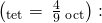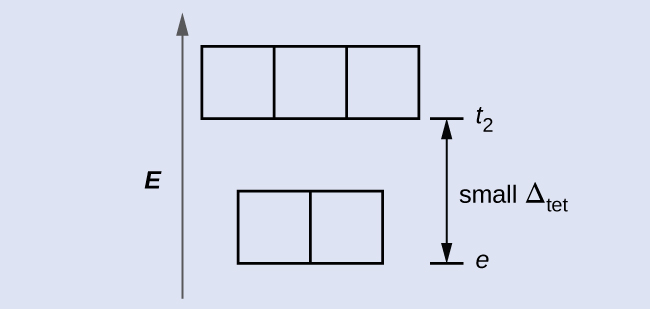Check Your Learning Explain how many unpaired electrons a tetrahedral d4 ion will have.

Answer:

4; because Δtet is small, all tetrahedral complexes are high spin and the electrons go into the t2 orbitals before pairing

The other common geometry is square planar. It is possible to consider a square planar geometry as an octahedral structure with a pair of trans ligands removed. The removed ligands are assumed to be on the z-axis. This changes the distribution of the d orbitals, as orbitals on or near the z-axis become more stable, and those on or near the x- or y-axes become less stable. This results in the octahedral t2g and the eg sets splitting and gives a more complicated pattern with no simple Δoct. The basic pattern is: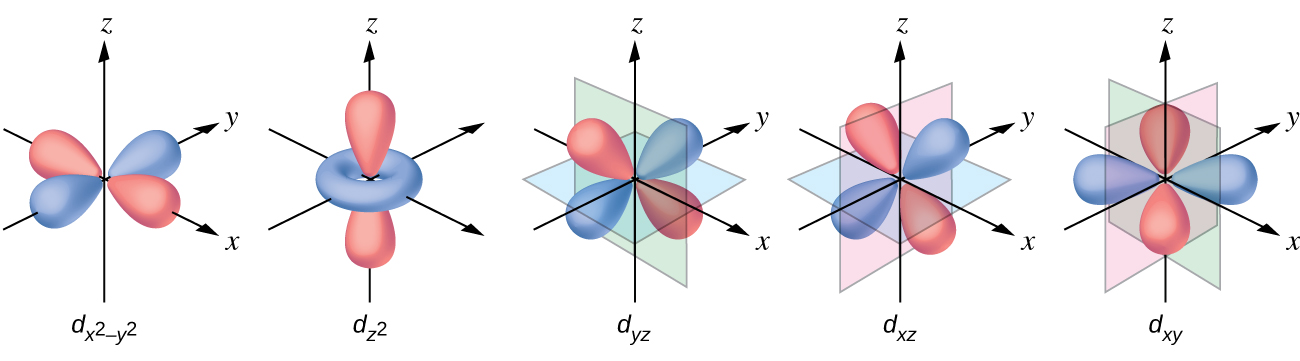### Magnetic Moments of Molecules and Ions

Experimental evidence of magnetic measurements supports the theory of high- and low-spin complexes. Remember that molecules such as O2 that contain unpaired electrons are paramagnetic. Paramagnetic substances are attracted to magnetic fields. Many transition metal complexes have unpaired electrons and hence are paramagnetic. Molecules such as N2 and ions such as Na+ and [Fe(CN)6]4− that contain no unpaired electrons are diamagnetic. Diamagnetic substances have a slight tendency to be repelled by magnetic fields.

When an electron in an atom or ion is unpaired, the magnetic moment due to its spin makes the entire atom or ion paramagnetic. The size of the magnetic moment of a system containing unpaired electrons is related directly to the number of such electrons: the greater the number of unpaired electrons, the larger the magnetic moment. Therefore, the observed magnetic moment is used to determine the number of unpaired electrons present. The measured magnetic moment of low-spin d6 [Fe(CN)6]4− confirms that iron is diamagnetic, whereas high-spin d6 [Fe(H2O)6]2+ has four unpaired electrons with a magnetic moment that confirms this arrangement.

### Colors of Transition Metal Complexes

When atoms or molecules absorb light at the proper frequency, their electrons are excited to higher-energy orbitals. For many main group atoms and molecules, the absorbed photons are in the ultraviolet range of the electromagnetic spectrum, which cannot be detected by the human eye. For coordination compounds, the energy difference between the d orbitals often allows photons in the visible range to be absorbed.

The human eye perceives a mixture of all the colors, in the proportions present in sunlight, as white light. Complementary colors, those located across from each other on a color wheel, are also used in color vision. The eye perceives a mixture of two complementary colors, in the proper proportions, as white light. Likewise, when a color is missing from white light, the eye sees its complement. For example, when red photons are absorbed from white light, the eyes see the color green. When violet photons are removed from white light, the eyes see lemon yellow. The blue color of the [Cu(NH3)4]2+ ion results because this ion absorbs orange and red light, leaving the complementary colors of blue and green ((Figure)).

(a) An object is black if it absorbs all colors of light. If it reflects all colors of light, it is white. An object has a color if it absorbs all colors except one, such as this yellow strip. The strip also appears yellow if it absorbs the complementary color from white light (in this case, indigo). (b) Complementary colors are located directly across from one another on the color wheel. (c) A solution of [Cu(NH3)4]2+ ions absorbs red and orange light, so the transmitted light appears as the complementary color, blue.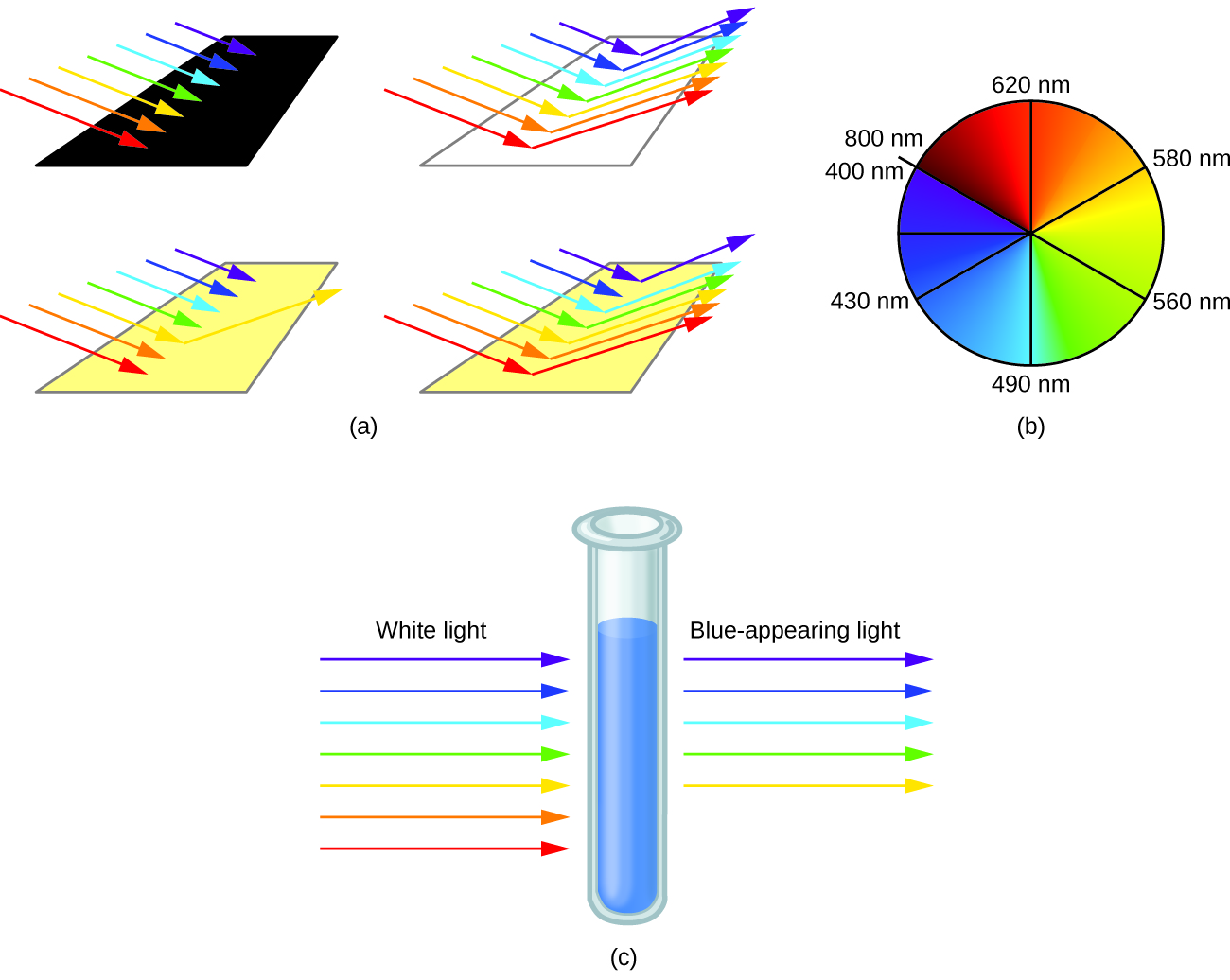Colors of Complexes The octahedral complex [Ti(H2O)6]3+ has a single d electron. To excite this electron from the ground state t2g orbital to the eg orbital, this complex absorbs light from 450 to 600 nm. The maximum absorbance corresponds to Δoct and occurs at 499 nm. Calculate the value of Δoct in Joules and predict what color the solution will appear.

Solution Using Planck’s equation (refer to the section on electromagnetic energy), we calculate: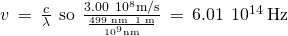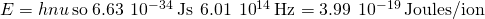Because the complex absorbs 600 nm (orange) through 450 (blue), the indigo, violet, and red wavelengths will be transmitted, and the complex will appear purple.

Check Your Learning A complex that appears green, absorbs photons of what wavelengths?

Answer:

red, 620–800 nm

Small changes in the relative energies of the orbitals that electrons are transitioning between can lead to drastic shifts in the color of light absorbed. Therefore, the colors of coordination compounds depend on many factors. As shown in (Figure), different aqueous metal ions can have different colors. In addition, different oxidation states of one metal can produce different colors, as shown for the vanadium complexes in the link below.

The partially filled d orbitals of the stable ions Cr3+(aq), Fe3+(aq), and Co2+(aq) (left, center and right, respectively) give rise to various colors. (credit: Sahar Atwa)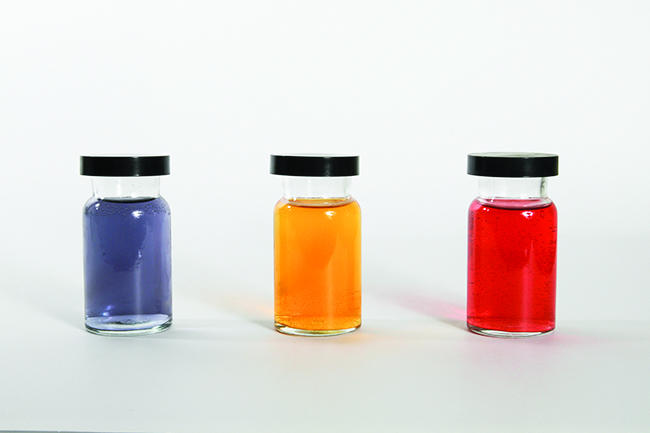The specific ligands coordinated to the metal center also influence the color of coordination complexes. For example, the iron(II) complex [Fe(H2O)6]SO4 appears blue-green because the high-spin complex absorbs photons in the red wavelengths ((Figure)). In contrast, the low-spin iron(II) complex K4[Fe(CN)6] appears pale yellow because it absorbs higher-energy violet photons.

Both (a) hexaaquairon(II) sulfate and (b) potassium hexacyanoferrate(II) contain d6 iron(II) octahedral metal centers, but they absorb photons in different ranges of the visible spectrum.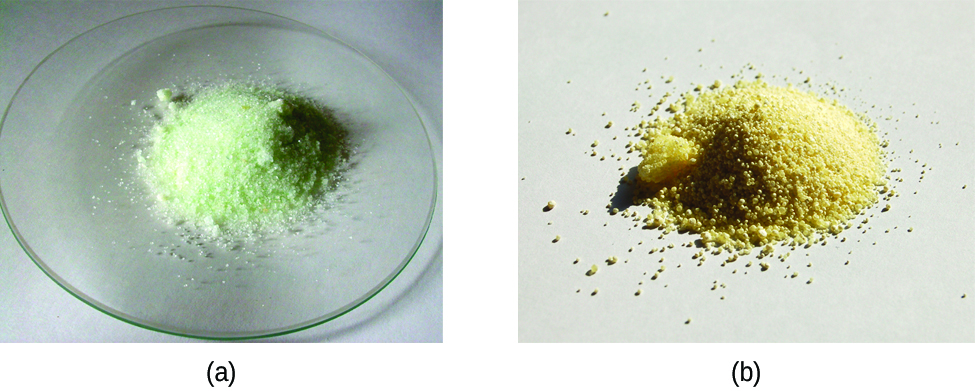In general, strong-field ligands cause a large split in the energies of d orbitals of the central metal atom (large Δoct). Transition metal coordination compounds with these ligands are yellow, orange, or red because they absorb higher-energy violet or blue light. On the other hand, coordination compounds of transition metals with weak-field ligands are often blue-green, blue, or indigo because they absorb lower-energy yellow, orange, or red light.

A coordination compound of the Cu+ ion has a d10 configuration, and all the eg orbitals are filled. To excite an electron to a higher level, such as the 4p orbital, photons of very high energy are necessary. This energy corresponds to very short wavelengths in the ultraviolet region of the spectrum. No visible light is absorbed, so the eye sees no change, and the compound appears white or colorless. A solution containing [Cu(CN)2], for example, is colorless. On the other hand, octahedral Cu2+ complexes have a vacancy in the eg orbitals, and electrons can be excited to this level. The wavelength (energy) of the light absorbed corresponds to the visible part of the spectrum, and Cu2+ complexes are almost always colored—blue, blue-green violet, or yellow ((Figure)). Although CFT successfully describes many properties of coordination complexes, molecular orbital explanations (beyond the introductory scope provided here) are required to understand fully the behavior of coordination complexes.

(a) Copper(I) complexes with d10 configurations such as CuI tend to be colorless, whereas (b) d9 copper(II) complexes such as Cu(NO3)2·5H2O are brightly colored.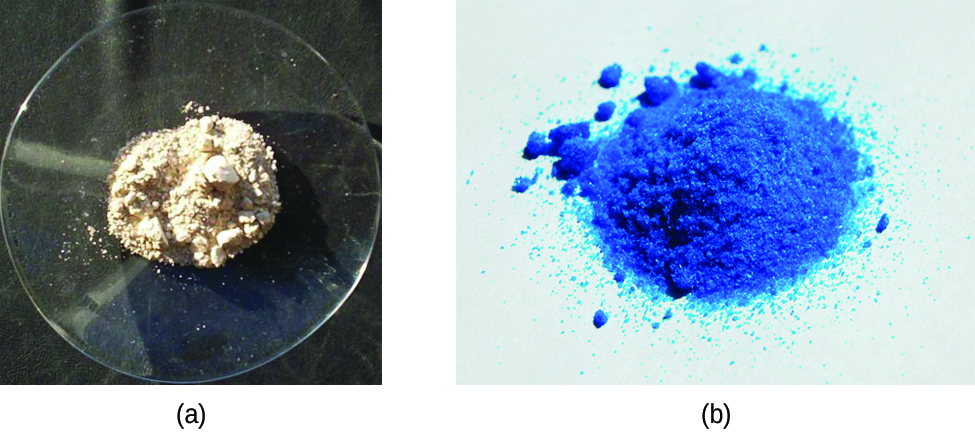### Key Concepts and Summary

Crystal field theory treats interactions between the electrons on the metal and the ligands as a simple electrostatic effect. The presence of the ligands near the metal ion changes the energies of the metal d orbitals relative to their energies in the free ion. Both the color and the magnetic properties of a complex can be attributed to this crystal field splitting. The magnitude of the splitting (Δoct) depends on the nature of the ligands bonded to the metal. Strong-field ligands produce large splitting and favor low-spin complexes, in which the t2g orbitals are completely filled before any electrons occupy the eg orbitals. Weak-field ligands favor formation of high-spin complexes. The t2g and the eg orbitals are singly occupied before any are doubly occupied.

### Chemistry End of Chapter Exercises

Determine the number of unpaired electrons expected for [Fe(NO2)6]3−and for [FeF6]3− in terms of crystal field theory.

Draw the crystal field diagrams for [Fe(NO2)6]4− and [FeF6]3−. State whether each complex is high spin or low spin, paramagnetic or diamagnetic, and compare Δoct to P for each complex.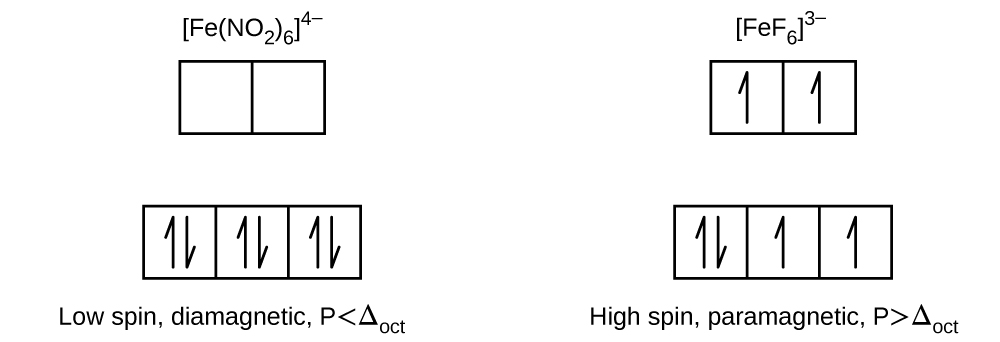Give the oxidation state of the metal, number of d electrons, and the number of unpaired electrons predicted for [Co(NH3)6]Cl3.

The solid anhydrous solid CoCl2 is blue in color. Because it readily absorbs water from the air, it is used as a humidity indicator to monitor if equipment (such as a cell phone) has been exposed to excessive levels of moisture. Predict what product is formed by this reaction, and how many unpaired electrons this complex will have.

[Co(H2O)6]Cl2 with three unpaired electrons.

Is it possible for a complex of a metal in the transition series to have six unpaired electrons? Explain.

How many unpaired electrons are present in each of the following?

(a) [CoF6]3− (high spin)

(b) [Mn(CN)6]3− (low spin)

(c) [Mn(CN)6]4− (low spin)

(d) [MnCl6]4− (high spin)

(e) [RhCl6]3− (low spin)

(a) 4; (b) 2; (c) 1; (d) 5; (e) 0

Explain how the diphosphate ion, [O3P−O−PO3]4−, can function as a water softener that prevents the precipitation of Fe2+ as an insoluble iron salt.

For complexes of the same metal ion with no change in oxidation number, the stability increases as the number of electrons in the t2g orbitals increases. Which complex in each of the following pairs of complexes is more stable?

(a) [Fe(H2O)6]2+ or [Fe(CN)6]4−

(b) [Co(NH3)6]3+ or [CoF6]3−

(c) [Mn(CN)6]4− or [MnCl6]4−

(a) [Fe(CN)6]4−; (b) [Co(NH3)6]3+; (c) [Mn(CN)6]4−

Trimethylphosphine, P(CH3)3, can act as a ligand by donating the lone pair of electrons on the phosphorus atom. If trimethylphosphine is added to a solution of nickel(II) chloride in acetone, a blue compound that has a molecular mass of approximately 270 g and contains 21.5% Ni, 26.0% Cl, and 52.5% P(CH3)3 can be isolated. This blue compound does not have any isomeric forms. What are the geometry and molecular formula of the blue compound?

Would you expect the complex [Co(en)3]Cl3 to have any unpaired electrons? Any isomers?

The complex does not have any unpaired electrons. The complex does not have any geometric isomers, but the mirror image is nonsuperimposable, so it has an optical isomer.

Would you expect the Mg3[Cr(CN)6]2 to be diamagnetic or paramagnetic? Explain your reasoning.

Would you expect salts of the gold(I) ion, Au+, to be colored? Explain.

No. Au+ has a complete 5d sublevel.

[CuCl4]2− is green. [Cu(H2O)6]2+is blue. Which absorbs higher-energy photons? Which is predicted to have a larger crystal field splitting?

### Glossary

crystal field splitting (Δoct)
difference in energy between the t2g and eg sets or t and e sets of orbitals
crystal field theory
model that explains the energies of the orbitals in transition metals in terms of electrostatic interactions with the ligands but does not include metal ligand bonding
eg orbitals
set of two d orbitals that are oriented on the Cartesian axes for coordination complexes; in octahedral complexes, they are higher in energy than the t2g orbitals
geometric isomers
isomers that differ in the way in which atoms are oriented in space relative to each other, leading to different physical and chemical properties
high-spin complex
complex in which the electrons maximize the total electron spin by singly populating all of the orbitals before pairing two electrons into the lower-energy orbitals
low-spin complex
complex in which the electrons minimize the total electron spin by pairing in the lower-energy orbitals before populating the higher-energy orbitals
pairing energy (P)
energy required to place two electrons with opposite spins into a single orbital
spectrochemical series
ranking of ligands according to the magnitude of the crystal field splitting they induce
strong-field ligand
ligand that causes larger crystal field splittings
t2g orbitals
set of three d orbitals aligned between the Cartesian axes for coordination complexes; in octahedral complexes, they are lowered in energy compared to the eg orbitals according to CFT
weak-field ligand
ligand that causes small crystal field splittings

## LicenseChemistry 2e by OSCRiceUniversity is licensed under a Creative Commons Attribution 4.0 International License, except where otherwise noted.